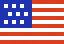New Swift-BAT/GUANO and IceCube Notice Types Available! See news and announcements

# GCN Circular 7862

Subject
Konus-Wind observation of GRB 080607
Date
2008-06-08T15:49:55Z (15 years ago)
From
Valentin Pal'shin at Ioffe Inst <val@mail.ioffe.ru>
``````S. Golenetskii, R.Aptekar, E. Mazets, V. Pal'shin, D. Frederiks, P.
Oleynik, M. Ulanov, D. Svinkin, and
T. Cline on behalf of the Konus-Wind team report:

The bright GRB 080607 (Swift-BAT trigger #313417: Mangano et al., GCN
7847, Stamatikos et al., GCN 7852) triggered Konus-Wind at T0=22043.336
s UT (06:07:23.336).

The burst light curve consists of the main part, which shows a
multipeaked structure with a duration of ~16 s, and the extended
emission, which shows many weak pulses up to ~T0+85 s in the soft energy
band (~18-70 keV).

As observed by Konus-Wind the burst
had a fluence of 8.93(-0.47, +0.52)x10^-5 erg/cm2,
and a 16-ms peak flux measured from T0+7.168 s
of (2.69 +/- 0.54)x10^-5 erg/cm2/s
(both in the 20 keV - 4 MeV energy range).

The time-integrated spectrum of the burst
(from T0 to T0+84.48 s) can be fitted (in the 20 keV - 4 MeV range)
by a power law with exponential cutoff model:
dN/dE ~ (E^alpha)*exp(-E*(2+alpha)/Ep)
with alpha = -1.08(-0.06, +0.07),
and Ep = 419(-38, +46) keV (chi2 = 78.8/73 dof).
Fitting by GRBM (Band) model yields:
the low-energy photon index is alpha = -1.06(-0.08, +0.09),
the high energy photon index beta < -2.28,
the peak energy Ep = 394(-54, +58) keV (chi2 = 77.2/72 dof).

The spectrum of the main part
(from T0 to T0+18.944 s) can be fitted (in the 20 keV - 4 MeV range)
by GRBM (Band) model for which:
the low-energy photon index is alpha = -0.76(-0.06, +0.07),
the high energy photon index beta = -2.57(-0.26, +0.18),
the peak energy Ep = (348 +/- 27) keV (chi2 = 99.6/72 dof).
The fluence of this part is (8.59 +/- 0.40)x10^-5 erg/cm2.

All the quoted errors are at the 90% confidence level.

Assuming z = 3.036 (Prochaska et al., GCN 7849) and a standard
cosmology model with H_0 = 70 km/s/Mpc, Omega_M = 0.27, Omega_\Lambda =
0.73, the isotropic energy release is E_iso = 1.87(-0.10, +0.11)x10^54
erg, the peak luminosity is (L_iso)_max = (2.27 +/- 0.46)x10^54 erg/s,
and Ep_rest ~1600 keV.
This isotropic energy release is one of the largest ever measured
(the current record holder is GRB 990123 for which the isotropic energy
release is ~2.8x10^54 erg), and this peak luminosity is the highest ever
reported.

The Konus-Wind light curve of this GRB is available